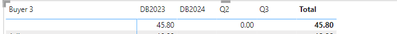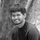cancel
Showing results for
Did you mean:Frequent Visitor

## If condition isn't working with all column

Hi Guys,

Am trying below IF formula and its not working with all column , can you please advise why or how can i solve it.1 ACCEPTED SOLUTIONSuper User

Hi,

Does this calculated column formula work

=F('Calender Test'[Quarter]="Q1","DB"&YEAR('Calender Test'[Date])+1,IF('Calender Test'[Quarter]="Q4","DB"&YEAR('Calender Test'[Date])+2,'Calender Test'[Quarter]))

Regards,
Ashish Mathur
http://www.ashishmathur.com
8 REPLIES 8Super User

Hi,

Does this calculated column formula work

=F('Calender Test'[Quarter]="Q1","DB"&YEAR('Calender Test'[Date])+1,IF('Calender Test'[Quarter]="Q4","DB"&YEAR('Calender Test'[Date])+2,'Calender Test'[Quarter]))

Regards,
Ashish Mathur
http://www.ashishmathur.comFrequent Visitor

Thank you Guyes i figured it out :).Frequent Visitor

Thank you Ashish it worked but its not showin correctly in the Matrix as below.
the right arrangment asper the date should be DB2023 Q2 Q3 DB2024. any recommendation ?Community Champion

what do you get if you try like:Frequent VisitorSuper User

I don't see anything wrong with the DAX but you don't need to use 'Calender Test'[Date].[Date], you can just use 'Calender Test'[Date].  Also, it's easier to use a SWITCH statement instead of nested IFs so it would look like this.

``````Internal Q =
SWITCH (
TRUE (),
'Calender Test'[Quarter] = "Q1", "DB" & FORMAT ( DATEADD ( 'Calender Test'[Date], 1, YEAR ), "YY" ),
'Calender Test'[Quarter] = "Q4", "DB" & FORMAT ( DATEADD ( 'Calender Test'[Date], 2, YEAR ), "YY" ),
'Calender Test'[Quarter]
)``````

What output are you trying to get?Frequent Visitor

am trying to replace all Q1 +2 row year to be shown as (1/1/2022 and Q1 to appear as DB24) in date columnSuper User

What is the expected result here?

Thanks,

Arul

Regards,
Arul
If I answered your question kindly mark my post as a solution and a kudo would be appreciated.